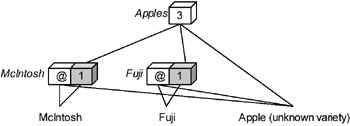# INCOMPLETE INFORMATION IN THE HIERARCHY

Some of the techniques for managing incomplete information in the base data presented earlier involve inserting incompleteness into the derived data. Unknown and imprecise values are the most common kinds of incomplete derived data. The techniques to handle incomplete derived data are essentially the same as those for handling incomplete base data, but some additional strategies exist for improving the responsiveness of queries on the incomplete data.

Suggest an alternative, complete query: A query on incomplete derived data can be automatically redirected to the "nearest" complete derived data (Dyreson, 1996; Pedersen et al., 2001). Figure 6 shows a fragment of a hierarchy for maintaining the count of the number of food items sold in a grocery store application. In the example, three apples are sold, but it is unknown how many Fuji and McIntosh apples are sold because the variety of one of the apples is incompletely known (the value of the variety attribute is the imprecise value "Apple," so the fact is effectively grouped higher in the hierarchy (Pedersen et al., 1999; Jagadish et al., 1999; Pourabbas & Rafanelli, 2000)).Figure 6: Suggesting an Alternative, Complete Query

To manage the incomplete base data, the base nodes are split into visible and hidden components. The hidden component is shaded in gray. It accurately counts the complete information for the base node. The visible component is shaded in white. Each visible component contains an unknown value indicating that some number of apples were sold, but it is unknown how many. A query for the number of Fuji apples cannot be satisfied since the number is unknown. The query should be redirected to the closest complete information. The closest complete information can be found by moving up the hierarchy, since the incompleteness inherent in imprecise and disjunctive values disappear at some coarse, imprecise category (sometimes only at the top). In the hierarchy shown in Figure 6, the closest complete information is found immediately above, at the "Apples" unit. The user is advised to ask that query to obtain a definite answer.

Computing min-max bounds: Incomplete derived data is often bounded by complete derived data above and below it in the hierarchy. For a count aggregate, if the information in a derived (or base) node is incomplete, the lower bound is the closest complete information below the node, whereas the upper bound is the closest complete information above it. In the hierarchy shown in Figure 6, the min-max bounds for Fuji apples are [1–3]. The hidden component shows that there is at least one Fuji apple. Above it in the hierarchy is the information that there are at most three apples. This is a crude upper bound that could be made tighter by discounting the known contribution of other apple varieties, e.g., since there is at least one McIntosh apple, the upper bound on Fuji apples is two (Dyreson, 1996).Multidimensional Databases: Problems and Solutions
ISBN: 1591400538
EAN: 2147483647
Year: 2003
Pages: 150